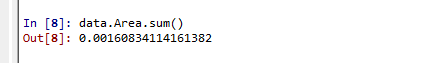# Area of slice : CellSize vs Integrate Variables

I need the area of my cell data as a variable to calculate area-weighted average values. To get that I am using Filter> CellSize > Compute Area. This gives me area on my cell data. I am exporting these values in csv files and this serves my purpose. I was calculating the total area summing all the cells. I got the total area here:Then I was cross-checking my values using IntegratVariables function. I got these area values from ingerateVariables :

These two values are not close. My understanding was these two values will be close. What I am missing?

What is the best way to get cell area ?